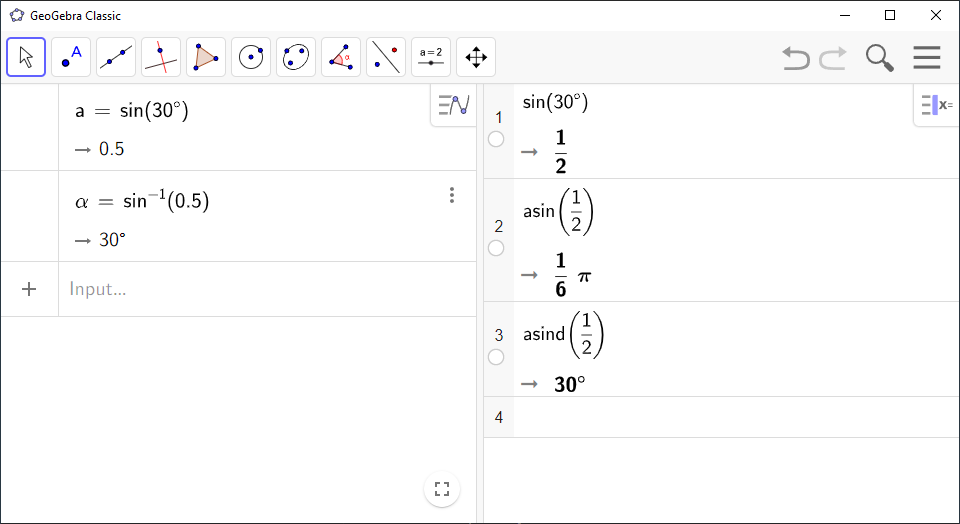# How to Use Trigonometric Functions in GeoGebra

`GeoGebra` has the built-in functions `sin(<x>)`, `cos(<x>)` and `tan(<x>)` for calculating the sine, the cosine and the tangent of angles.

`GeoGebra` uses the names `asin(<x>)` and `acos(<x>)`, for the inverse functions ${\mathrm{sin}}^{-1}\left(x\right)$ and ${\mathrm{cos}}^{-1}\left(x\right)$ for $x$ in the interval $\phantom{\rule{-0.17em}{0ex}}\left[-1,1\right]$, and `atan(<x>)` for ${\mathrm{tan}}^{-1}\left(x\right)$ for any $x$. Use the inverse functions to calculate the angles of a right triangle when you already know two or all the side lengths, by typing the expression directly in `Algebra View` or `CAS`.

When you input angles measured in degrees, you have to use the ${}^{\circ }$ symbol (keyboard shortcut `alt+o`).

Note! If you try to insert a value less than $-1$ or greater than 1, `GeoGebra` will return a number containing the letter $i$. This indicates that you have received a complex solution. Unless you know that complex solutions are fine for your problem, this solution indicates that you input something wrong.

Note! In some versions of `GeoGebra`, angles in `Algebra View` are by default measured in degrees, while angles in `CAS` are measured in radians. If you want `CAS` to use degrees, use the commands `asind(<x>)`, `acosd(<x>)`, and `atand(<x>)`, which are the regular commands with a `d` at the end of the command name.

You can also get the answer in degrees quickly by dividing your answer by ${}^{\circ }$ or by the string `deg`, such as `asin(<x>)/${}^{\circ }$`. It may seem strange to divide by ${}^{\circ }$, but it works!

`GeoGebra` Instruction 1

### TrigonometricExpressionsin`Algebra View`

1.
Open `Algebra View` under`View` in`Menu`.
2.
Type the expression and press `Enter`.

`GeoGebra` Instruction 2

### TrigonometricExpressionsin`CAS`

1.
Open `CAS` under`View` in`Menu`.
2.
Type the expression. If you press `Enter` you get the exact answer. If you press `Symbolic Evaluation`you also get the exact answer. If an exact value does not exist, `GeoGebra` writes the answer in radians. Therefore, press `Numeric Evaluation`to get the answer as a decimal number.